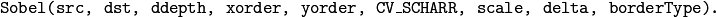# Image Filtering¶

Functions and classes described in this section are used to perform various linear or non-linear filtering operations on 2D images (represented as Mat()‘s). It means that for each pixel location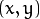in the source image (normally, rectangular), its neighborhood is considered and used to compute the response. In case of a linear filter, it is a weighted sum of pixel values. In case of morphological operations, it is the minimum or maximum values, and so on. The computed response is stored in the destination image at the same location. It means that the output image will be of the same size as the input image. Normally, the functions support multi-channel arrays, in which case every channel is processed independently. Therefore, the output image will also have the same number of channels as the input one.

Another common feature of the functions and classes described in this section is that, unlike simple arithmetic functions, they need to extrapolate values of some non-existing pixels. For example, if you want to smooth an image using a Gaussian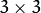filter, then, when processing the left-most pixels in each row, you need pixels to the left of them, that is, outside of the image. You can let these pixels be the same as the left-most image pixels (“replicated border” extrapolation method), or assume that all the non-existing pixels are zeros (“contant border” extrapolation method), and so on. OpenCV enables you to specify the extrapolation method. For details, see the function borderInterpolate() and discussion of the borderType parameter in various functions below.

## BaseColumnFilter¶

class BaseColumnFilter

Base class for filters with single-column kernels.

class BaseColumnFilter
{
public:
virtual ~BaseColumnFilter();

// To be overriden by the user.
//
// runs a filtering operation on the set of rows,
// "dstcount + ksize - 1" rows on input,
// "dstcount" rows on output,
// each input and output row has "width" elements
// the filtered rows are written into "dst" buffer.
virtual void operator()(const uchar** src, uchar* dst, int dststep,
int dstcount, int width) = 0;
// resets the filter state (may be needed for IIR filters)
virtual void reset();

int ksize; // the aperture size
int anchor; // position of the anchor point,
// normally not used during the processing
};


The class BaseColumnFilter is a base class for filtering data using single-column kernels. Filtering does not have to be a linear operation. In general, it could be written as follows: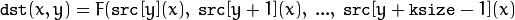where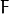is a filtering function but, as it is represented as a class, it can produce any side effects, memorize previously processed data, and so on. The class only defines an interface and is not used directly. Instead, there are several functions in OpenCV (and you can add more) that return pointers to the derived classes that implement specific filtering operations. Those pointers are then passed to the FilterEngine constructor. While the filtering operation interface uses the uchar type, a particular implementation is not limited to 8-bit data.

## BaseFilter¶

class BaseFilter

Base class for 2D image filters.

class BaseFilter
{
public:
virtual ~BaseFilter();

// To be overriden by the user.
//
// runs a filtering operation on the set of rows,
// "dstcount + ksize.height - 1" rows on input,
// "dstcount" rows on output,
// each input row has "(width + ksize.width-1)*cn" elements
// each output row has "width*cn" elements.
// the filtered rows are written into "dst" buffer.
virtual void operator()(const uchar** src, uchar* dst, int dststep,
int dstcount, int width, int cn) = 0;
// resets the filter state (may be needed for IIR filters)
virtual void reset();
Size ksize;
Point anchor;
};


The class BaseFilter is a base class for filtering data using 2D kernels. Filtering does not have to be a linear operation. In general, it could be written as follows: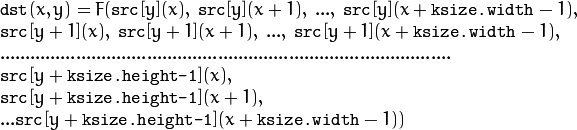whereis a filtering function. The class only defines an interface and is not used directly. Instead, there are several functions in OpenCV (and you can add more) that return pointers to the derived classes that implement specific filtering operations. Those pointers are then passed to the FilterEngine constructor. While the filtering operation interface uses the uchar type, a particular implementation is not limited to 8-bit data.

## BaseRowFilter¶

class BaseRowFilter

Base class for filters with single-row kernels.

class BaseRowFilter
{
public:
virtual ~BaseRowFilter();

// To be overriden by the user.
//
// runs filtering operation on the single input row
// of "width" element, each element is has "cn" channels.
// the filtered row is written into "dst" buffer.
virtual void operator()(const uchar* src, uchar* dst,
int width, int cn) = 0;
int ksize, anchor;
};


The class BaseRowFilter is a base class for filtering data using single-row kernels. Filtering does not have to be a linear operation. In general, it could be written as follows: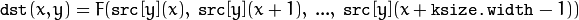whereis a filtering function. The class only defines an interface and is not used directly. Instead, there are several functions in OpenCV (and you can add more) that return pointers to the derived classes that implement specific filtering operations. Those pointers are then passed to the FilterEngine constructor. While the filtering operation interface uses the uchar type, a particular implementation is not limited to 8-bit data.

## FilterEngine¶

class FilterEngine

Generic image filtering class.

class FilterEngine
{
public:
// empty constructor
FilterEngine();
// builds a 2D non-separable filter (!_filter2D.empty()) or
// a separable filter (!_rowFilter.empty() && !_columnFilter.empty())
// the input data type will be "srcType", the output data type will be "dstType",
// the intermediate data type is "bufType".
// _rowBorderType and _columnBorderType determine how the image
// will be extrapolated beyond the image boundaries.
// _borderValue is only used when _rowBorderType and/or _columnBorderType
// == BORDER_CONSTANT
FilterEngine(const Ptr<BaseFilter>& _filter2D,
const Ptr<BaseRowFilter>& _rowFilter,
const Ptr<BaseColumnFilter>& _columnFilter,
int srcType, int dstType, int bufType,
int _rowBorderType=BORDER_REPLICATE,
int _columnBorderType=-1, // use _rowBorderType by default
const Scalar& _borderValue=Scalar());
virtual ~FilterEngine();
// separate function for the engine initialization
void init(const Ptr<BaseFilter>& _filter2D,
const Ptr<BaseRowFilter>& _rowFilter,
const Ptr<BaseColumnFilter>& _columnFilter,
int srcType, int dstType, int bufType,
int _rowBorderType=BORDER_REPLICATE, int _columnBorderType=-1,
const Scalar& _borderValue=Scalar());
// starts filtering of the ROI in an image of size "wholeSize".
// returns the starting y-position in the source image.
virtual int start(Size wholeSize, Rect roi, int maxBufRows=-1);
// alternative form of start that takes the image
// itself instead of "wholeSize". Set isolated to true to pretend that
// there are no real pixels outside of the ROI
// (so that the pixels are extrapolated using the specified border modes)
virtual int start(const Mat& src, const Rect& srcRoi=Rect(0,0,-1,-1),
bool isolated=false, int maxBufRows=-1);
// processes the next portion of the source image,
// "srcCount" rows starting from "src" and
// stores the results in "dst".
// returns the number of produced rows
virtual int proceed(const uchar* src, int srcStep, int srcCount,
uchar* dst, int dstStep);
// higher-level function that processes the whole
// ROI or the whole image with a single call
virtual void apply( const Mat& src, Mat& dst,
const Rect& srcRoi=Rect(0,0,-1,-1),
Point dstOfs=Point(0,0),
bool isolated=false);
bool isSeparable() const { return filter2D.empty(); }
// how many rows from the input image are not yet processed
int remainingInputRows() const;
// how many output rows are not yet produced
int remainingOutputRows() const;
...
// the starting and the ending rows in the source image
int startY, endY;

// pointers to the filters
Ptr<BaseFilter> filter2D;
Ptr<BaseRowFilter> rowFilter;
Ptr<BaseColumnFilter> columnFilter;
};


The class FilterEngine can be used to apply an arbitrary filtering operation to an image. It contains all the necessary intermediate buffers, computes extrapolated values of the “virtual” pixels outside of the image, and so on. Pointers to the initialized FilterEngine instances are returned by various create*Filter functions (see below) and they are used inside high-level functions such as filter2D(), erode(), dilate(), and others. Thus, the class plays a key role in many of OpenCV filtering functions.

This class makes it easier to combine filtering operations with other operations, such as color space conversions, thresholding, arithmetic operations, and others. By combining several operations together you can get much better performance because your data will stay in cache. For example, see below the implementation of the Laplace operator for floating-point images, which is a simplified implementation of Laplacian() :

void laplace_f(const Mat& src, Mat& dst)
{
CV_Assert( src.type() == CV_32F );
dst.create(src.size(), src.type());

// get the derivative and smooth kernels for d2I/dx2.
// for d2I/dy2 consider using the same kernels, just swapped
Mat kd, ks;
getSobelKernels( kd, ks, 2, 0, ksize, false, ktype );

// process 10 source rows at once
int DELTA = std::min(10, src.rows);
Ptr<FilterEngine> Fxx = createSeparableLinearFilter(src.type(),
dst.type(), kd, ks, Point(-1,-1), 0, borderType, borderType, Scalar() );
Ptr<FilterEngine> Fyy = createSeparableLinearFilter(src.type(),
dst.type(), ks, kd, Point(-1,-1), 0, borderType, borderType, Scalar() );

int y = Fxx->start(src), dsty = 0, dy = 0;
Fyy->start(src);
const uchar* sptr = src.data + y*src.step;

// allocate the buffers for the spatial image derivatives;
// the buffers need to have more than DELTA rows, because at the
// last iteration the output may take max(kd.rows-1,ks.rows-1)
// rows more than the input.
Mat Ixx( DELTA + kd.rows - 1, src.cols, dst.type() );
Mat Iyy( DELTA + kd.rows - 1, src.cols, dst.type() );

// inside the loop always pass DELTA rows to the filter
// (note that the "proceed" method takes care of possibe overflow, since
// it was given the actual image height in the "start" method)
// on output you can get:
//  * < DELTA rows (initial buffer accumulation stage)
//  * = DELTA rows (settled state in the middle)
//  * > DELTA rows (when the input image is over, generate
//                  "virtual" rows using the border mode and filter them)
// this variable number of output rows is dy.
// dsty is the current output row.
// sptr is the pointer to the first input row in the portion to process
for( ; dsty < dst.rows; sptr += DELTA*src.step, dsty += dy )
{
Fxx->proceed( sptr, (int)src.step, DELTA, Ixx.data, (int)Ixx.step );
dy = Fyy->proceed( sptr, (int)src.step, DELTA, d2y.data, (int)Iyy.step );
if( dy > 0 )
{
Mat dstripe = dst.rowRange(dsty, dsty + dy);
}
}
}


If you do not need that much control of the filtering process, you can simply use the FilterEngine::apply method. The method is implemented as follows:

void FilterEngine::apply(const Mat& src, Mat& dst,
const Rect& srcRoi, Point dstOfs, bool isolated)
{
// check matrix types
CV_Assert( src.type() == srcType && dst.type() == dstType );

// handle the "whole image" case
Rect _srcRoi = srcRoi;
if( _srcRoi == Rect(0,0,-1,-1) )
_srcRoi = Rect(0,0,src.cols,src.rows);

// check if the destination ROI is inside dst.
// and FilterEngine::start will check if the source ROI is inside src.
CV_Assert( dstOfs.x >= 0 && dstOfs.y >= 0 &&
dstOfs.x + _srcRoi.width <= dst.cols &&
dstOfs.y + _srcRoi.height <= dst.rows );

// start filtering
int y = start(src, _srcRoi, isolated);

// process the whole ROI. Note that "endY - startY" is the total number
// of the source rows to process
// (including the possible rows outside of srcRoi but inside the source image)
proceed( src.data + y*src.step,
(int)src.step, endY - startY,
dst.data + dstOfs.y*dst.step +
dstOfs.x*dst.elemSize(), (int)dst.step );
}


Unlike the earlier versions of OpenCV, now the filtering operations fully support the notion of image ROI, that is, pixels outside of the ROI but inside the image can be used in the filtering operations. For example, you can take a ROI of a single pixel and filter it. This will be a filter response at that particular pixel. However, it is possible to emulate the old behavior by passing isolated=false to FilterEngine::start or FilterEngine::apply . You can pass the ROI explicitly to FilterEngine::apply or construct new matrix headers:

// compute dI/dx derivative at src(x,y)

// method 1:
// form a matrix header for a single value
float val1 = 0;
Mat dst1(1,1,CV_32F,&val1);

Ptr<FilterEngine> Fx = createDerivFilter(CV_32F, CV_32F,
1, 0, 3, BORDER_REFLECT_101);
Fx->apply(src, Rect(x,y,1,1), Point(), dst1);

// method 2:
// form a matrix header for a single value
float val2 = 0;
Mat dst2(1,1,CV_32F,&val2);

Mat pix_roi(src, Rect(x,y,1,1));
Sobel(pix_roi, dst2, dst2.type(), 1, 0, 3, 1, 0, BORDER_REFLECT_101);

printf("method1 =


Explore the data types. As it was mentioned in the BaseFilter description, the specific filters can process data of any type, despite that Base*Filter::operator() only takes uchar pointers and no information about the actual types. To make it all work, the following rules are used:

• In case of separable filtering, FilterEngine::rowFilter is applied first. It transforms the input image data (of type srcType ) to the intermediate results stored in the internal buffers (of type bufType ). Then, these intermediate results are processed as single-channel data with FilterEngine::columnFilter and stored in the output image (of type dstType ). Thus, the input type for rowFilter is srcType and the output type is bufType . The input type for columnFilter is CV_MAT_DEPTH(bufType) and the output type is CV_MAT_DEPTH(dstType) .
• In case of non-separable filtering, bufType must be the same as srcType . The source data is copied to the temporary buffer, if needed, and then just passed to FilterEngine::filter2D . That is, the input type for filter2D is srcType (= bufType ) and the output type is dstType .

## bilateralFilter¶

Applies the bilateral filter to an image.

C++: void bilateralFilter(InputArray src, OutputArray dst, int d, double sigmaColor, double sigmaSpace, int borderType=BORDER_DEFAULT )
Python: cv2.bilateralFilter(src, d, sigmaColor, sigmaSpace[, dst[, borderType]]) → dst
Parameters: src – Source 8-bit or floating-point, 1-channel or 3-channel image. dst – Destination image of the same size and type as src . d – Diameter of each pixel neighborhood that is used during filtering. If it is non-positive, it is computed from sigmaSpace . sigmaColor – Filter sigma in the color space. A larger value of the parameter means that farther colors within the pixel neighborhood (see sigmaSpace ) will be mixed together, resulting in larger areas of semi-equal color. sigmaSpace – Filter sigma in the coordinate space. A larger value of the parameter means that farther pixels will influence each other as long as their colors are close enough (see sigmaColor ). When d>0 , it specifies the neighborhood size regardless of sigmaSpace . Otherwise, d is proportional to sigmaSpace .

The function applies bilateral filtering to the input image, as described in http://www.dai.ed.ac.uk/CVonline/LOCAL_COPIES/MANDUCHI1/Bilateral_Filtering.html bilateralFilter can reduce unwanted noise very well while keeping edges fairly sharp. However, it is very slow compared to most filters.

Sigma values: For simplicity, you can set the 2 sigma values to be the same. If they are small (< 10), the filter will not have much effect, whereas if they are large (> 150), they will have a very strong effect, making the image look “cartoonish”.

Filter size: Large filters (d > 5) are very slow, so it is recommended to use d=5 for real-time applications, and perhaps d=9 for offline applications that need heavy noise filtering.

This filter does not work inplace.

## blur¶

Smoothes an image using the normalized box filter.

C++: void blur(InputArray src, OutputArray dst, Size ksize, Point anchor=Point(-1,-1), int borderType=BORDER_DEFAULT )
Python: cv2.blur(src, ksize[, dst[, anchor[, borderType]]]) → dst
Parameters: src – Source image. dst – Destination image of the same size and type as src . ksize – Smoothing kernel size. anchor – Anchor point. The default value Point(-1,-1) means that the anchor is at the kernel center. borderType – Border mode used to extrapolate pixels outside of the image.

The function smoothes an image using the kernel: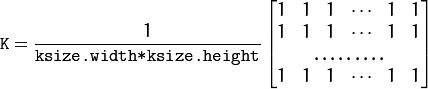The call blur(src, dst, ksize, anchor, borderType) is equivalent to boxFilter(src, dst, src.type(), anchor, true, borderType) .

## borderInterpolate¶

Computes the source location of an extrapolated pixel.

C++: int borderInterpolate(int p, int len, int borderType)
Python: cv2.borderInterpolate(p, len, borderType) → retval
Parameters: p – 0-based coordinate of the extrapolated pixel along one of the axes, likely <0 or >= len . len – Length of the array along the corresponding axis. borderType – Border type, one of the BORDER_* , except for BORDER_TRANSPARENT and BORDER_ISOLATED . When borderType==BORDER_CONSTANT , the function always returns -1, regardless of p and len .

The function computes and returns the coordinate of a donor pixel corresponding to the specified extrapolated pixel when using the specified extrapolation border mode. For example, if you use BORDER_WRAP mode in the horizontal direction, BORDER_REFLECT_101 in the vertical direction and want to compute value of the “virtual” pixel Point(-5, 100) in a floating-point image img , it looks like:

float val = img.at<float>(borderInterpolate(100, img.rows, BORDER_REFLECT_101),
borderInterpolate(-5, img.cols, BORDER_WRAP));


Normally, the function is not called directly. It is used inside FilterEngine and copyMakeBorder() to compute tables for quick extrapolation.

## boxFilter¶

Smoothes an image using the box filter.

C++: void boxFilter(InputArray src, OutputArray dst, int ddepth, Size ksize, Point anchor=Point(-1,-1), bool normalize=true, int borderType=BORDER_DEFAULT )
Python: cv2.boxFilter(src, ddepth, ksize[, dst[, anchor[, normalize[, borderType]]]]) → dst
Parameters: src – Source image. dst – Destination image of the same size and type as src . ksize – Smoothing kernel size. anchor – Anchor point. The default value Point(-1,-1) means that the anchor is at the kernel center. normalize – Flag specifying whether the kernel is normalized by its area or not. borderType – Border mode used to extrapolate pixels outside of the image.

The function smoothes an image using the kernel: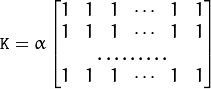where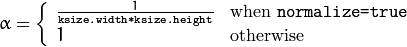Unnormalized box filter is useful for computing various integral characteristics over each pixel neighborhood, such as covariance matrices of image derivatives (used in dense optical flow algorithms, and so on). If you need to compute pixel sums over variable-size windows, use integral() .

## buildPyramid¶

Constructs the Gaussian pyramid for an image.

C++: void buildPyramid(InputArray src, OutputArrayOfArrays dst, int maxlevel)
Parameters: src – Source image. Check pyrDown() for the list of supported types. dst – Destination vector of maxlevel+1 images of the same type as src . dst will be the same as src . dst is the next pyramid layer, a smoothed and down-sized src , and so on. maxlevel – 0-based index of the last (the smallest) pyramid layer. It must be non-negative.

The function constructs a vector of images and builds the Gaussian pyramid by recursively applying pyrDown() to the previously built pyramid layers, starting from dst==src .

## copyMakeBorder¶

Forms a border around an image.

C++: void copyMakeBorder(InputArray src, OutputArray dst, int top, int bottom, int left, int right, int borderType, const Scalar& value=Scalar() )
Python: cv2.copyMakeBorder(src, top, bottom, left, right, borderType[, dst[, value]]) → dst
C: void cvCopyMakeBorder(const CvArr* src, CvArr* dst, CvPoint offset, int bordertype, CvScalar value=cvScalarAll(0) )
Python: cv.CopyMakeBorder(src, dst, offset, bordertype, value=(0, 0, 0, 0)) → None
Parameters: src – Source image. dst – Destination image of the same type as src and the size Size(src.cols+left+right, src.rows+top+bottom) . top – bottom – left – right – Parameter specifying how many pixels in each direction from the source image rectangle to extrapolate. For example, top=1, bottom=1, left=1, right=1 mean that 1 pixel-wide border needs to be built. borderType – Border type. See borderInterpolate() for details. value – Border value if borderType==BORDER_CONSTANT .

The function copies the source image into the middle of the destination image. The areas to the left, to the right, above and below the copied source image will be filled with extrapolated pixels. This is not what FilterEngine or filtering functions based on it do (they extrapolate pixels on-fly), but what other more complex functions, including your own, may do to simplify image boundary handling.

The function supports the mode when src is already in the middle of dst . In this case, the function does not copy src itself but simply constructs the border, for example:

// let border be the same in all directions
int border=2;
// constructs a larger image to fit both the image and the border
Mat gray_buf(rgb.rows + border*2, rgb.cols + border*2, rgb.depth());
// select the middle part of it w/o copying data
Mat gray(gray_canvas, Rect(border, border, rgb.cols, rgb.rows));
// convert image from RGB to grayscale
cvtColor(rgb, gray, CV_RGB2GRAY);
// form a border in-place
copyMakeBorder(gray, gray_buf, border, border,
border, border, BORDER_REPLICATE);
// now do some custom filtering ...
...


## createBoxFilter¶

Returns a box filter engine.

C++: Ptr<FilterEngine> createBoxFilter(int srcType, int dstType, Size ksize, Point anchor=Point(-1,-1), bool normalize=true, int borderType=BORDER_DEFAULT)
C++: Ptr<BaseRowFilter> getRowSumFilter(int srcType, int sumType, int ksize, int anchor=-1)
C++: Ptr<BaseColumnFilter> getColumnSumFilter(int sumType, int dstType, int ksize, int anchor=-1, double scale=1)
Parameters: srcType – Source image type. sumType – Intermediate horizontal sum type that must have as many channels as srcType . dstType – Destination image type that must have as many channels as srcType . ksize – Aperture size. anchor – Anchor position with the kernel. Negative values mean that the anchor is at the kernel center. normalize – Flag specifying whether the sums are normalized or not. See boxFilter() for details. scale – Another way to specify normalization in lower-level getColumnSumFilter . borderType – Border type to use. See borderInterpolate() .

The function is a convenience function that retrieves the horizontal sum primitive filter with getRowSumFilter() , vertical sum filter with getColumnSumFilter() , constructs new FilterEngine , and passes both of the primitive filters there. The constructed filter engine can be used for image filtering with normalized or unnormalized box filter.

The function itself is used by blur() and boxFilter() .

## createDerivFilter¶

Returns an engine for computing image derivatives.

C++: Ptr<FilterEngine> createDerivFilter(int srcType, int dstType, int dx, int dy, int ksize, int borderType=BORDER_DEFAULT )
Parameters: srcType – Source image type. dstType – Destination image type that must have as many channels as srcType . dx – Derivative order in respect of x. dy – Derivative order in respect of y. ksize – Aperture size See getDerivKernels() . borderType – Border type to use. See borderInterpolate() .

The function createDerivFilter() is a small convenience function that retrieves linear filter coefficients for computing image derivatives using getDerivKernels() and then creates a separable linear filter with createSeparableLinearFilter() . The function is used by Sobel() and Scharr() .

## createGaussianFilter¶

Returns an engine for smoothing images with the Gaussian filter.

C++: Ptr<FilterEngine> createGaussianFilter(int type, Size ksize, double sigmaX, double sigmaY=0, int borderType=BORDER_DEFAULT)
Parameters: type – Source and destination image type. ksize – Aperture size. See getGaussianKernel() . sigmaX – Gaussian sigma in the horizontal direction. See getGaussianKernel() . sigmaY – Gaussian sigma in the vertical direction. If 0, then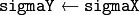. borderType – Border type to use. See borderInterpolate() .

The function createGaussianFilter() computes Gaussian kernel coefficients and then returns a separable linear filter for that kernel. The function is used by GaussianBlur() . Note that while the function takes just one data type, both for input and output, you can pass this limitation by calling getGaussianKernel() and then createSeparableLinearFilter() directly.

## createLinearFilter¶

Creates a non-separable linear filter engine.

C++: Ptr<FilterEngine> createLinearFilter(int srcType, int dstType, InputArray kernel, Point _anchor=Point(-1,-1), double delta=0, int rowBorderType=BORDER_DEFAULT, int columnBorderType=-1, const Scalar& borderValue=Scalar())
C++: Ptr<BaseFilter> getLinearFilter(int srcType, int dstType, InputArray kernel, Point anchor=Point(-1,-1), double delta=0, int bits=0)
Parameters: srcType – Source image type. dstType – Destination image type that must have as many channels as srcType . kernel – 2D array of filter coefficients. anchor – Anchor point within the kernel. Special value Point(-1,-1) means that the anchor is at the kernel center. delta – Value added to the filtered results before storing them. bits – Number of the fractional bits. the parameter is used when the kernel is an integer matrix representing fixed-point filter coefficients. rowBorderType – Pixel extrapolation method in the vertical direction. For details, see borderInterpolate(). columnBorderType – Pixel extrapolation method in the horizontal direction. borderValue – Border vaule used in case of a constant border.

The function returns a pointer to a 2D linear filter for the specified kernel, the source array type, and the destination array type. The function is a higher-level function that calls getLinearFilter and passes the retrieved 2D filter to the FilterEngine constructor.

## createMorphologyFilter¶

Creates an engine for non-separable morphological operations.

C++: Ptr<FilterEngine> createMorphologyFilter(int op, int type, InputArray element, Point anchor=Point(-1,-1), int rowBorderType=BORDER_CONSTANT, int columnBorderType=-1, const Scalar& borderValue=morphologyDefaultBorderValue())
C++: Ptr<BaseFilter> getMorphologyFilter(int op, int type, InputArray element, Point anchor=Point(-1,-1))
C++: Ptr<BaseRowFilter> getMorphologyRowFilter(int op, int type, int esize, int anchor=-1)
C++: Ptr<BaseColumnFilter> getMorphologyColumnFilter(int op, int type, int esize, int anchor=-1)
C++: Scalar morphologyDefaultBorderValue()
Parameters: op – Morphology operation ID, MORPH_ERODE or MORPH_DILATE . type – Input/output image type. element – 2D 8-bit structuring element for a morphological operation. Non-zero elements indicate the pixels that belong to the element. esize – Horizontal or vertical structuring element size for separable morphological operations. anchor – Anchor position within the structuring element. Negative values mean that the anchor is at the kernel center. rowBorderType – Pixel extrapolation method in the vertical direction. For details, see borderInterpolate(). columnBorderType – Pixel extrapolation method in the horizontal direction. borderValue – Border value in case of a constant border. The default value, morphologyDefaultBorderValue , has a special meaning. It is transformed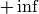for the erosion and to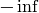for the dilation, which means that the minimum (maximum) is effectively computed only over the pixels that are inside the image.

The functions construct primitive morphological filtering operations or a filter engine based on them. Normally it is enough to use createMorphologyFilter() or even higher-level erode(), dilate() , or morphologyEx() . Note that createMorphologyFilter() analyzes the structuring element shape and builds a separable morphological filter engine when the structuring element is square.

## createSeparableLinearFilter¶

Creates an engine for a separable linear filter.

C++: Ptr<FilterEngine> createSeparableLinearFilter(int srcType, int dstType, InputArray rowKernel, InputArray columnKernel, Point anchor=Point(-1,-1), double delta=0, int rowBorderType=BORDER_DEFAULT, int columnBorderType=-1, const Scalar& borderValue=Scalar())
C++: Ptr<BaseColumnFilter> getLinearColumnFilter(int bufType, int dstType, InputArray columnKernel, int anchor, int symmetryType, double delta=0, int bits=0)
C++: Ptr<BaseRowFilter> getLinearRowFilter(int srcType, int bufType, InputArray rowKernel, int anchor, int symmetryType)
Parameters: srcType – Source array type. dstType – Destination image type that must have as many channels as srcType . bufType – Intermediate buffer type that must have as many channels as srcType . rowKernel – Coefficients for filtering each row. columnKernel – Coefficients for filtering each column. anchor – Anchor position within the kernel. Negative values mean that anchor is positioned at the aperture center. delta – Value added to the filtered results before storing them. bits – Number of the fractional bits. The parameter is used when the kernel is an integer matrix representing fixed-point filter coefficients. rowBorderType – Pixel extrapolation method in the vertical direction. For details, see borderInterpolate(). columnBorderType – Pixel extrapolation method in the horizontal direction. borderValue – Border value used in case of a constant border. symmetryType – Type of each row and column kernel. See getKernelType() .

The functions construct primitive separable linear filtering operations or a filter engine based on them. Normally it is enough to use createSeparableLinearFilter() or even higher-level sepFilter2D() . The function createMorphologyFilter() is smart enough to figure out the symmetryType for each of the two kernels, the intermediate bufType and, if filtering can be done in integer arithmetics, the number of bits to encode the filter coefficients. If it does not work for you, it is possible to call getLinearColumnFilter,getLinearRowFilter directly and then pass them to the FilterEngine constructor.

## dilate¶

Dilates an image by using a specific structuring element.

C++: void dilate(InputArray src, OutputArray dst, InputArray element, Point anchor=Point(-1,-1), int iterations=1, int borderType=BORDER_CONSTANT, const Scalar& borderValue=morphologyDefaultBorderValue() )
Python: cv2.dilate(src, kernel[, dst[, anchor[, iterations[, borderType[, borderValue]]]]]) → dst
C: void cvDilate(const CvArr* src, CvArr* dst, IplConvKernel* element=NULL, int iterations=1 )
Python: cv.Dilate(src, dst, element=None, iterations=1) → None
Parameters: src – Source image. dst – Destination image of the same size and type as src . element – Structuring element used for dilation. If element=Mat() , a 3 x 3 rectangular structuring element is used. anchor – Position of the anchor within the element. The default value (-1, -1) means that the anchor is at the element center. iterations – Number of times dilation is applied. borderType – Pixel extrapolation method. See borderInterpolate() for details. borderValue – Border value in case of a constant border. The default value has a special meaning. See createMorphologyFilter() for details.

The function dilates the source image using the specified structuring element that determines the shape of a pixel neighborhood over which the maximum is taken: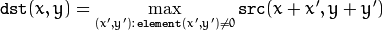The function supports the in-place mode. Dilation can be applied several ( iterations ) times. In case of multi-channel images, each channel is processed independently.

## erode¶

Erodes an image by using a specific structuring element.

C++: void erode(InputArray src, OutputArray dst, InputArray element, Point anchor=Point(-1,-1), int iterations=1, int borderType=BORDER_CONSTANT, const Scalar& borderValue=morphologyDefaultBorderValue() )
Python: cv2.erode(src, kernel[, dst[, anchor[, iterations[, borderType[, borderValue]]]]]) → dst
C: void cvErode(const CvArr* src, CvArr* dst, IplConvKernel* element=NULL, int iterations=1)
Python: cv.Erode(src, dst, element=None, iterations=1) → None
Parameters: src – Source image. dst – Destination image of the same size and type as src . element – Structuring element used for erosion. If element=Mat() , a 3 x 3 rectangular structuring element is used. anchor – Position of the anchor within the element. The default value (-1, -1) means that the anchor is at the element center. iterations – Number of times erosion is applied. borderType – Pixel extrapolation method. See borderInterpolate() for details. borderValue – Border value in case of a constant border. The default value has a special meaning. See createMorphologyFilter() for details.

The function erodes the source image using the specified structuring element that determines the shape of a pixel neighborhood over which the minimum is taken: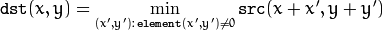The function supports the in-place mode. Erosion can be applied several ( iterations ) times. In case of multi-channel images, each channel is processed independently.

## filter2D¶

Convolves an image with the kernel.

C++: void filter2D(InputArray src, OutputArray dst, int ddepth, InputArray kernel, Point anchor=Point(-1,-1), double delta=0, int borderType=BORDER_DEFAULT )
Python: cv2.filter2D(src, ddepth, kernel[, dst[, anchor[, delta[, borderType]]]]) → dst
C: void cvFilter2D(const CvArr* src, CvArr* dst, const CvMat* kernel, CvPoint anchor=cvPoint(-1, -1))
Python: cv.Filter2D(src, dst, kernel, anchor=(-1, -1)) → None
Parameters: src – Source image. dst – Destination image of the same size and the same number of channels as src . ddepth – Desired depth of the destination image. If it is negative, it will be the same as src.depth() . kernel – Convolution kernel (or rather a correlation kernel), a single-channel floating point matrix. If you want to apply different kernels to different channels, split the image into separate color planes using split() and process them individually. anchor – Anchor of the kernel that indicates the relative position of a filtered point within the kernel. The anchor should lie within the kernel. The special default value (-1,-1) means that the anchor is at the kernel center. delta – Optional value added to the filtered pixels before storing them in dst . borderType – Pixel extrapolation method. See borderInterpolate() for details.

The function applies an arbitrary linear filter to an image. In-place operation is supported. When the aperture is partially outside the image, the function interpolates outlier pixel values according to the specified border mode.

The function does actually compute correlation, not the convolution: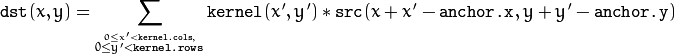That is, the kernel is not mirrored around the anchor point. If you need a real convolution, flip the kernel using flip() and set the new anchor to (kernel.cols - anchor.x - 1, kernel.rows - anchor.y - 1) .

The function uses the DFT-based algorithm in case of sufficiently large kernels (~11 x 11 or larger) and the direct algorithm (that uses the engine retrieved by createLinearFilter() ) for small kernels.

## GaussianBlur¶

Smoothes an image using a Gaussian filter.

C++: void GaussianBlur(InputArray src, OutputArray dst, Size ksize, double sigmaX, double sigmaY=0, int borderType=BORDER_DEFAULT )
Python: cv2.GaussianBlur(src, ksize, sigma1[, dst[, sigma2[, borderType]]]) → dst
Parameters: src – Source image. dst – Destination image of the same size and type as src . ksize – Gaussian kernel size. ksize.width and ksize.height can differ but they both must be positive and odd. Or, they can be zero’s and then they are computed from sigma* . sigmaX – Gaussian kernel standard deviation in X direction. sigmaY – Gaussian kernel standard deviation in Y direction. If sigmaY is zero, it is set to be equal to sigmaX . If both sigmas are zeros, they are computed from ksize.width and ksize.height , respectively. See getGaussianKernel() for details. To fully control the result regardless of possible future modifications of all this semantics, it is recommended to specify all of ksize , sigmaX , and sigmaY . borderType – Pixel extrapolation method. See borderInterpolate() for details.

The function convolves the source image with the specified Gaussian kernel. In-place filtering is supported.

## getDerivKernels¶

Returns filter coefficients for computing spatial image derivatives.

C++: void getDerivKernels(OutputArray kx, OutputArray ky, int dx, int dy, int ksize, bool normalize=false, int ktype=CV_32F )
Python: cv2.getDerivKernels(dx, dy, ksize[, kx[, ky[, normalize[, ktype]]]]) → kx, ky
Parameters: kx – Output matrix of row filter coefficients. It has the type ktype . ky – Output matrix of column filter coefficients. It has the type ktype . dx – Derivative order in respect of x. dy – Derivative order in respect of y. ksize – Aperture size. It can be CV_SCHARR , 1, 3, 5, or 7. normalize – Flag indicating whether to normalize (scale down) the filter coefficients or not. Theoretically, the coefficients should have the denominator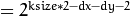. If you are going to filter floating-point images, you are likely to use the normalized kernels. But if you compute derivatives of an 8-bit image, store the results in a 16-bit image, and wish to preserve all the fractional bits, you may want to set normalize=false . ktype – Type of filter coefficients. It can be CV_32f or CV_64F .

The function computes and returns the filter coefficients for spatial image derivatives. When ksize=CV_SCHARR , the Scharrkernels are generated (see Scharr() ). Otherwise, Sobel kernels are generated (see Sobel() ). The filters are normally passed to sepFilter2D() or to createSeparableLinearFilter() .

## getGaussianKernel¶

Returns Gaussian filter coefficients.

C++: Mat getGaussianKernel(int ksize, double sigma, int ktype=CV_64F )
Python: cv2.getGaussianKernel(ksize, sigma[, ktype]) → retval
Parameters: ksize – Aperture size. It should be odd (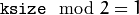) and positive. sigma – Gaussian standard deviation. If it is non-positive, it is computed from ksize as sigma = 0.3*((ksize-1)*0.5 - 1) + 0.8 . ktype – Type of filter coefficients. It can be CV_32f or CV_64F .

The function computes and returns the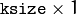matrix of Gaussian filter coefficients: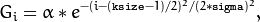where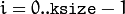and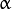is the scale factor chosen so that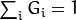.

Two of such generated kernels can be passed to sepFilter2D() or to createSeparableLinearFilter(). Those functions automatically recognize smoothing kernels (a symmetrical kernel with sum of weights equal to 1) and handle them accordingly. You may also use the higher-level GaussianBlur().

## getKernelType¶

Returns the kernel type.

C++: int getKernelType(InputArray kernel, Point anchor)
Parameters: kernel – 1D array of the kernel coefficients to analyze. anchor – Anchor position within the kernel.

The function analyzes the kernel coefficients and returns the corresponding kernel type:

• KERNEL_GENERAL The kernel is generic. It is used when there is no any type of symmetry or other properties.
• KERNEL_SYMMETRICAL The kernel is symmetrical: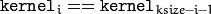, and the anchor is at the center.
• KERNEL_ASYMMETRICAL The kernel is asymmetrical: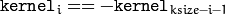, and the anchor is at the center.
• KERNEL_SMOOTH All the kernel elements are non-negative and summed to 1. For example, the Gaussian kernel is both smooth kernel and symmetrical, so the function returns KERNEL_SMOOTH | KERNEL_SYMMETRICAL .
• KERNEL_INTEGER All the kernel coefficients are integer numbers. This flag can be combined with KERNEL_SYMMETRICAL or KERNEL_ASYMMETRICAL .

## getStructuringElement¶

Returns a structuring element of the specified size and shape for morphological operations.

C++: Mat getStructuringElement(int shape, Size ksize, Point anchor=Point(-1,-1))
Python: cv2.getStructuringElement(shape, ksize[, anchor]) → retval
C: IplConvKernel* cvCreateStructuringElementEx(int cols, int rows, int anchorX, int anchorY, int shape, int* values=NULL )
Python: cv.CreateStructuringElementEx(cols, rows, anchorX, anchorY, shape, values=None) → kernel
Parameters: shape – Element shape that could be one of the following: MORPH_RECT - a rectangular structuring element: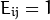MORPH_ELLIPSE - an elliptic structuring element, that is, a filled ellipse inscribed into the rectangle Rect(0, 0, esize.width, 0.esize.height) MORPH_CROSS - a cross-shaped structuring element: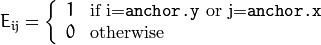CV_SHAPE_CUSTOM - custom structuring element (OpenCV 1.x API) ksize – Size of the structuring element. cols – Width of the structuring element rows – Height of the structuring element anchor – Anchor position within the element. The default value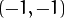means that the anchor is at the center. Note that only the shape of a cross-shaped element depends on the anchor position. In other cases the anchor just regulates how much the result of the morphological operation is shifted. anchorX – x-coordinate of the anchor anchorY – y-coordinate of the anchor values – integer array of cols*rows elements that specifies the custom shape of the structuring element, when shape=CV_SHAPE_CUSTOM.

The function constructs and returns the structuring element that can be further passed to createMorphologyFilter(), erode(), dilate() or morphologyEx() . But you can also construct an arbitrary binary mask yourself and use it as the structuring element.

Note

When using OpenCV 1.x C API, the created structuring element IplConvKernel* element must be released in the end using cvReleaseStructuringElement(&element).

## medianBlur¶

Smoothes an image using the median filter.

C++: void medianBlur(InputArray src, OutputArray dst, int ksize)
Python: cv2.medianBlur(src, ksize[, dst]) → dst
Parameters: src – Source 1-, 3-, or 4-channel image. When ksize is 3 or 5, the image depth should be CV_8U , CV_16U , or CV_32F . For larger aperture sizes, it can only be CV_8U . dst – Destination array of the same size and type as src . ksize – Aperture linear size. It must be odd and greater than 1, for example: 3, 5, 7 ...

The function smoothes an image using the median filter with the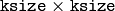aperture. Each channel of a multi-channel image is processed independently. In-place operation is supported.

## morphologyEx¶

C++: void morphologyEx(InputArray src, OutputArray dst, int op, InputArray element, Point anchor=Point(-1,-1), int iterations=1, int borderType=BORDER_CONSTANT, const Scalar& borderValue=morphologyDefaultBorderValue() )
Python: cv2.morphologyEx(src, op, kernel[, dst[, anchor[, iterations[, borderType[, borderValue]]]]]) → dst
C: void cvMorphologyEx(const CvArr* src, CvArr* dst, CvArr* temp, IplConvKernel* element, int operation, int iterations=1 )
Python: cv.MorphologyEx(src, dst, temp, element, operation, iterations=1) → None
Parameters: src – Source image. dst – Destination image of the same size and type as src . element – Structuring element. op – Type of a morphological operation that can be one of the following: MORPH_OPEN - an opening operation MORPH_CLOSE - a closing operation MORPH_GRADIENT - a morphological gradient MORPH_TOPHAT - “top hat” MORPH_BLACKHAT - “black hat” iterations – Number of times erosion and dilation are applied. borderType – Pixel extrapolation method. See borderInterpolate() for details. borderValue – Border value in case of a constant border. The default value has a special meaning. See createMorphologyFilter() for details.

The function can perform advanced morphological transformations using an erosion and dilation as basic operations.

Opening operation: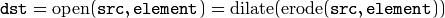Closing operation: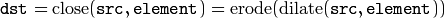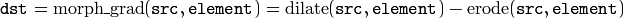“Top hat”: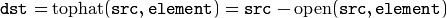“Black hat”: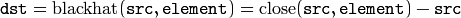Any of the operations can be done in-place.

## Laplacian¶

Calculates the Laplacian of an image.

C++: void Laplacian(InputArray src, OutputArray dst, int ddepth, int ksize=1, double scale=1, double delta=0, int borderType=BORDER_DEFAULT )
Python: cv2.Laplacian(src, ddepth[, dst[, ksize[, scale[, delta[, borderType]]]]]) → dst
C: void cvLaplace(const CvArr* src, CvArr* dst, int ksize=3)
Python: cv.Laplace(src, dst, ksize=3) → None
Parameters: src – Source image. dst – Destination image of the same size and the same number of channels as src . ddepth – Desired depth of the destination image. ksize – Aperture size used to compute the second-derivative filters. See getDerivKernels() for details. The size must be positive and odd. scale – Optional scale factor for the computed Laplacian values. By default, no scaling is applied. See getDerivKernels() for details. delta – Optional delta value that is added to the results prior to storing them in dst . borderType – Pixel extrapolation method. See borderInterpolate() for details.

The function calculates the Laplacian of the source image by adding up the second x and y derivatives calculated using the Sobel operator: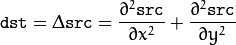This is done when ksize > 1 . When ksize == 1 , the Laplacian is computed by filtering the image with the followingaperture: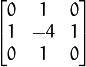## pyrDown¶

Smoothes an image and downsamples it.

C++: void pyrDown(InputArray src, OutputArray dst, const Size& dstsize=Size())
Python: cv2.pyrDown(src[, dst[, dstsize]]) → dst
C: void cvPyrDown(const CvArr* src, CvArr* dst, int filter=CV_GAUSSIAN_5x5 )
Python: cv.PyrDown(src, dst, filter=CV_GAUSSIAN_5X5) → None
Parameters: src – Source image. dst – Destination image. It has the specified size and the same type as src . dstsize – Size of the destination image. By default, it is computed as Size((src.cols+1)/2, (src.rows+1)/2) . But in any case, the following conditions should be satisfied: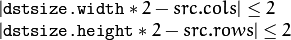The function performs the downsampling step of the Gaussian pyramid construction. First, it convolves the source image with the kernel: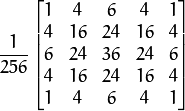Then, it downsamples the image by rejecting even rows and columns.

## pyrUp¶

Upsamples an image and then smoothes it.

C++: void pyrUp(InputArray src, OutputArray dst, const Size& dstsize=Size())
Python: cv2.pyrUp(src[, dst[, dstsize]]) → dst
Parameters: src – Source image. dst – Destination image. It has the specified size and the same type as src . dstsize – Size of the destination image. By default, it is computed as Size(src.cols*2, (src.rows*2) . But in any case, the following conditions should be satisfied: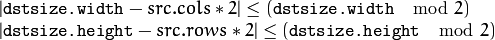The function performs the upsampling step of the Gaussian pyramid construction though it can actually be used to construct the Laplacian pyramid. First, it upsamples the source image by injecting even zero rows and columns and then convolves the result with the same kernel as in pyrDown() multiplied by 4.

## pyrMeanShiftFiltering¶

Performs initial step of meanshift segmentation of an image.

C++: void pyrMeanShiftFiltering(InputArray src, OutputArray dst, double sp, double sr, int maxLevel=1, TermCriteria termcrit=TermCriteria(TermCriteria::MAX_ITER+TermCriteria::EPS,5,1) )
Python: cv2.pyrMeanShiftFiltering(src, sp, sr[, dst[, maxLevel[, termcrit]]]) → dst
C: void cvPyrMeanShiftFiltering(const CvArr* src, CvArr* dst, double sp, double sr, int max_level=1, CvTermCriteria termcrit=cvTermCriteria(CV_TERMCRIT_ITER+CV_TERMCRIT_EPS,5,1))
Python: cv.PyrMeanShiftFiltering(src, dst, sp, sr, maxLevel=1, termcrit=(CV_TERMCRIT_ITER+CV_TERMCRIT_EPS, 5, 1)) → None
Parameters: src – The source 8-bit, 3-channel image. dst – The destination image of the same format and the same size as the source. sp – The spatial window radius. sr – The color window radius. maxLevel – Maximum level of the pyramid for the segmentation. termcrit – Termination criteria: when to stop meanshift iterations.

The function implements the filtering stage of meanshift segmentation, that is, the output of the function is the filtered “posterized” image with color gradients and fine-grain texture flattened. At every pixel (X,Y) of the input image (or down-sized input image, see below) the function executes meanshift iterations, that is, the pixel (X,Y) neighborhood in the joint space-color hyperspace is considered: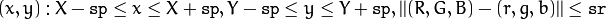where (R,G,B) and (r,g,b) are the vectors of color components at (X,Y) and (x,y), respectively (though, the algorithm does not depend on the color space used, so any 3-component color space can be used instead). Over the neighborhood the average spatial value (X',Y') and average color vector (R',G',B') are found and they act as the neighborhood center on the next iteration: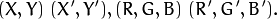After the iterations over, the color components of the initial pixel (that is, the pixel from where the iterations started) are set to the final value (average color at the last iteration):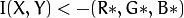When maxLevel > 0, the gaussian pyramid of maxLevel+1 levels is built, and the above procedure is run on the smallest layer first. After that, the results are propagated to the larger layer and the iterations are run again only on those pixels where the layer colors differ by more than sr from the lower-resolution layer of the pyramid. That makes boundaries of color regions sharper. Note that the results will be actually different from the ones obtained by running the meanshift procedure on the whole original image (i.e. when maxLevel==0).

## sepFilter2D¶

Applies a separable linear filter to an image.

C++: void sepFilter2D(InputArray src, OutputArray dst, int ddepth, InputArray rowKernel, InputArray columnKernel, Point anchor=Point(-1,-1), double delta=0, int borderType=BORDER_DEFAULT )
Python: cv2.sepFilter2D(src, ddepth, kernelX, kernelY[, dst[, anchor[, delta[, borderType]]]]) → dst
Parameters: src – Source image. dst – Destination image of the same size and the same number of channels as src . ddepth – Destination image depth. rowKernel – Coefficients for filtering each row. columnKernel – Coefficients for filtering each column. anchor – Anchor position within the kernel. The default value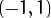means that the anchor is at the kernel center. delta – Value added to the filtered results before storing them. borderType – Pixel extrapolation method. See borderInterpolate() for details.

The function applies a separable linear filter to the image. That is, first, every row of src is filtered with the 1D kernel rowKernel . Then, every column of the result is filtered with the 1D kernel columnKernel . The final result shifted by delta is stored in dst .

## Smooth¶

Smooths the image in one of several ways.

C: void cvSmooth(const CvArr* src, CvArr* dst, int smoothtype=CV_GAUSSIAN, int param1=3, int param2=0, double param3=0, double param4=0)
Python: cv.Smooth(src, dst, smoothtype=CV_GAUSSIAN, param1=3, param2=0, param3=0, param4=0) → None
Parameters: src – The source image dst – The destination image smoothtype – Type of the smoothing: CV_BLUR_NO_SCALE linear convolution with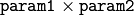box kernel (all 1’s). If you want to smooth different pixels with different-size box kernels, you can use the integral image that is computed using integral() CV_BLUR linear convolution withbox kernel (all 1’s) with subsequent scaling by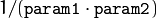CV_GAUSSIAN linear convolution with aGaussian kernel CV_MEDIAN median filter with a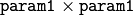square aperture CV_BILATERAL bilateral filter with asquare aperture, color sigma= param3 and spatial sigma= param4 . If param1=0 , the aperture square side is set to cvRound(param4*1.5)*2+1 . Information about bilateral filtering can be found at http://www.dai.ed.ac.uk/CVonline/LOCAL_COPIES/MANDUCHI1/Bilateral_Filtering.html param1 – The first parameter of the smoothing operation, the aperture width. Must be a positive odd number (1, 3, 5, ...) param2 – The second parameter of the smoothing operation, the aperture height. Ignored by CV_MEDIAN and CV_BILATERAL methods. In the case of simple scaled/non-scaled and Gaussian blur if param2 is zero, it is set to param1 . Otherwise it must be a positive odd number. param3 – In the case of a Gaussian parameter this parameter may specify Gaussian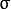(standard deviation). If it is zero, it is calculated from the kernel size: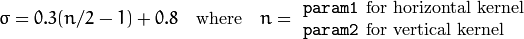Using standard sigma for small kernels (to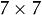) gives better speed. If param3 is not zero, while param1 and param2 are zeros, the kernel size is calculated from the sigma (to provide accurate enough operation).

The function smooths an image using one of several methods. Every of the methods has some features and restrictions listed below:

• Blur with no scaling works with single-channel images only and supports accumulation of 8-bit to 16-bit format (similar to Sobel() and Laplacian()) and 32-bit floating point to 32-bit floating-point format.
• Simple blur and Gaussian blur support 1- or 3-channel, 8-bit and 32-bit floating point images. These two methods can process images in-place.
• Median and bilateral filters work with 1- or 3-channel 8-bit images and can not process images in-place.

Note

The function is now obsolete. Use GaussianBlur(), blur(), medianBlur() or bilateralFilter().

## Sobel¶

Calculates the first, second, third, or mixed image derivatives using an extended Sobel operator.

C++: void Sobel(InputArray src, OutputArray dst, int ddepth, int xorder, int yorder, int ksize=3, double scale=1, double delta=0, int borderType=BORDER_DEFAULT )
Python: cv2.Sobel(src, ddepth, dx, dy[, dst[, ksize[, scale[, delta[, borderType]]]]]) → dst
C: void cvSobel(const CvArr* src, CvArr* dst, int xorder, int yorder, int apertureSize=3 )
Python: cv.Sobel(src, dst, xorder, yorder, apertureSize=3) → None
Parameters: src – Source image. dst – Destination image of the same size and the same number of channels as src . ddepth – Destination image depth. xorder – Order of the derivative x. yorder – Order of the derivative y. ksize – Size of the extended Sobel kernel. It must be 1, 3, 5, or 7. scale – Optional scale factor for the computed derivative values. By default, no scaling is applied. See getDerivKernels() for details. delta – Optional delta value that is added to the results prior to storing them in dst . borderType – Pixel extrapolation method. See borderInterpolate() for details.

In all cases except one, theseparable kernel is used to calculate the derivative. When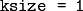, the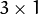or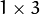kernel is used (that is, no Gaussian smoothing is done). ksize = 1 can only be used for the first or the second x- or y- derivatives.

There is also the special value ksize = CV_SCHARR (-1) that corresponds to theScharr filter that may give more accurate results than theSobel. The Scharr aperture is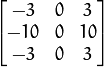for the x-derivative, or transposed for the y-derivative.

The function calculates an image derivative by convolving the image with the appropriate kernel:The Sobel operators combine Gaussian smoothing and differentiation, so the result is more or less resistant to the noise. Most often, the function is called with ( xorder = 1, yorder = 0, ksize = 3) or ( xorder = 0, yorder = 1, ksize = 3) to calculate the first x- or y- image derivative. The first case corresponds to a kernel of: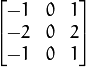The second case corresponds to a kernel of: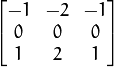## Scharr¶

Calculates the first x- or y- image derivative using Scharr operator.

C++: void Scharr(InputArray src, OutputArray dst, int ddepth, int xorder, int yorder, double scale=1, double delta=0, int borderType=BORDER_DEFAULT )
Python: cv2.Scharr(src, ddepth, dx, dy[, dst[, scale[, delta[, borderType]]]]) → dst
Parameters: src – Source image. dst – Destination image of the same size and the same number of channels as src . ddepth – Destination image depth. xorder – Order of the derivative x. yorder – Order of the derivative y. scale – Optional scale factor for the computed derivative values. By default, no scaling is applied. See getDerivKernels() for details. delta – Optional delta value that is added to the results prior to storing them in dst . borderType – Pixel extrapolation method. See borderInterpolate() for details.

The function computes the first x- or y- spatial image derivative using the Scharr operator. The call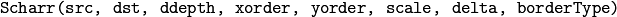is equivalent to